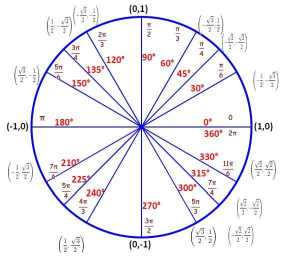AM
Animated Mathematics

### Svenska# Introduction to the Unit Circle

The unit circle is a circle with radius r=1 and the point P(x,y) can be anywhere on the circumference. The line from the origon to the point P forms an angle with the horizontal x-axis. The unit circle allows for a formal definition for $$sin \theta$$ and $$cos \theta$$ for angles $$\theta > 90$$. The x coordinate of P is $$cos \theta$$ and the y coordinate of P is $$sin \theta$$ , i.e. $$x=cos \theta$$ and $$y=sin \theta$$. That is the fundamental concept behind trigonometry. Students often find this confusing at first because it is so simple.

The cos and sin functions of a scientific calculator accepts an angle and returns the x and y coordinates of the point P respectively. You can test your calculator with the unit circle on the left where the numbers have been rounded to 4 decimal places. The calculator should be in degrees mode. From the unit circle and the definition of cos and sin it follows that:

cos(0)=1, cos(180)=-1, cos(90)=0, and cos(270)=0.

### The range of the cosine function is: $$-1\leq cos \theta \leq 1$$

sin(0)=0, sin(180)=0, sin(90)=1, and sin(270)=-1

### The range of the sine function is: $$-1\leq sin \theta \leq 1$$

In most textbooks $$\theta$$ is replaced by x, and note this x is not the x-axis of the unit circle, this can be another source of confusion.

## Solve for the angle $$\theta$$

A common problem involving the unit circle is, solve for the angle $$\theta$$ given $$cos \theta =k$$ or $$sin \theta=k$$ where $$-1\leq k \leq 1$$ and $$0\leq \theta \leq 360$$ .

Try the question before pressing to view the solution

# Summary

P(x,y) is a point on the circumference of the unit circle where $$x=cos \theta$$ and $$y=sin \theta$$, this is the formal defintion for the sine and cosine of angles that are greater than 90 degrees.

The solutions to $$cos \theta =k$$ can be found by first visualizing the intersections of the vertical line x=k and the unit circle. The first solution can be found using the calculators arcos function. The other solution is then found by reflecting the angle through the x-axis.

The solutions to $$sin \theta =k$$ can be found by first visualizing the intersections of the horizontal line y=k and the unit circle. The other solution is then found by reflecting the angle through the y-axis.

Understand the above and you have made a great start to the unit circle.